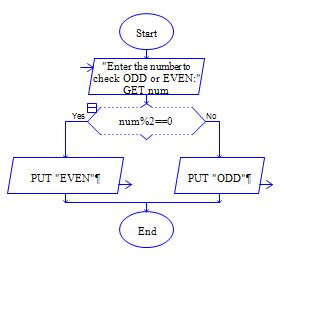# ODD or Even in Python

Python Program to get a number num and check whether num is odd or even?

Sample Input 1:

45

Sample Output 1:

ODD

Sample Input 2:

56

Sample Output 2:

EVEN

#### Flow Chart DesignStrongly recommended to Solve it on your own, Don't directly go to the solution given below.

#### Program or Solution

``` num1=int(input("Enter your number:")) if(num1%2==0): print("{} is even".format(num1)) else: print("{} is odd".format(num1)) ```

#### Program Explanation

Get input num from user using input() method check whether the remainder of num divided by 2 is equal to 0 using if statement.

if it is 0, then print num is even using print() method.

Else print num is odd using print() method.